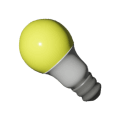Canada electricity sources | BCHydro Power Smart for Schools
Energy

# Sources of electricity in Canada

What are the main sources of electricity in Canada?9Duration
30 minsType
Slideshow

## Overview

Discuss and share thoughts on electricity generation in Canada using mathematical evidence from other sources and representations to support or refine ideas. Students use this mathematical evidence to refine their thinking about electricity generation in the country.

### Instructions

#### What you'll need

• "Electricity in Canada sources" handout
• "Identifying the main idea" worksheet
• "What’s the main idea?" slides
• Digital projector and screen

1. Organize your students into small groups and show slide 2 of the "What’s the main idea?" slides. Ask groups to suggest what the main idea of the image might be, encouraging them to use mathematical evidence to support their thinking.
2. Invite groups to share their decisions and thinking. As students share, prompt them to suggest how they identified the main idea.
3. Provide each student with a copy of the "Identifying the main idea" worksheet, and ask students to work on their own to suggest what the main idea might be. Remind them to record any mathematical evidence that supports their initial ideas.
4. Display slide 4 and briefly explain to students that they’ll examine four sources for mathematical evidence that could be used to support or refine their initial thinking about the main idea of the original image.
5. Provide each group with a copy of the "Electricity in Canada sources" handout. Show slide 5, and ask groups to suggest what mathematical evidence the source might provide.
6. Prompt students to note these ideas on their worksheet in the appropriate column for source 1.
7. Next, guide students’ attention to the right-hand column of the chart, and ask them to note how the mathematical evidence could be used to support or refine their initial ideas about the image presented at the beginning of this activity.
8. Ask groups to repeat this process with the remaining sources. Consider showing the slides that feature each source (slides 6-8).
9. After groups have examined each source, invite groups to share the ideas from their worksheets with the class. As groups share, encourage them to suggest which source provided the most useful mathematical evidence.
10. Prompt students to revisit their initial thinking about the image. Ask students to use the mathematical evidence from the sources to refine their initial ideas. Conclude the activity by inviting students to share their ideas with the class.

### Modify or extend this activity

#### Modifications

If students require additional support identifying the mathematical evidence in the sources, consider asking:

• For source 1 (slide 5): Which is most helpful: calculating the total usage of lights in Canada, determining the ratio of the number of lights to usage of lights, or finding the fraction of usage of ranges of lights to total usage in Canada?
• For source 2 (slide 6): Which is most helpful: calculating the value of each of the types of power generated, or the ratio of hydropower to natural gas?
• For source 3 (slide 7): Which is most helpful: determining the ratio of hydropower generation to the total generation, or determining the ratio of British Columbia’s electricity generation to Ontario’s electricity generation?
• For source 4 (slide 8): Which is most helpful: creating a tally of the different sizes of blue dots, or creating a ratio of the blue dots to the black dots?

#### Extensions

• Invite students to rework the initial image to more effectively deliver the main idea.

### Curriculum Fit

#### Big ideas

• Analyzing the validity, reliability, and representation of data enables us to compare and interpret

#### Content

• Statistics in society: analyzing a given set of data (and/or its representation) and identifying potential problems related to bias, use of language, ethics, cost, time and timing, privacy, or cultural sensitivity

#### Curricular competencies

##### Reasoning and analyzing
• Use reasoning and logic to explore, analyze, and apply mathematical ideas
• Use tools or technology to explore and create patterns and relationships, and test conjectures
##### Understanding and solving
• Apply multiple strategies to solve problems in both abstract and contextualized situations
• Develop, demonstrate, and apply mathematical understanding through play, inquiry, and problem solving
##### Communicating and representing
• Use mathematical vocabulary and language to contribute to mathematical discussions
• Explain and justify mathematical ideas and decisions
• Communicate mathematical thinking in many ways
• Represent mathematical ideas in concrete, pictorial, and symbolic forms
##### Connecting and reflecting
• Reflect on mathematical thinking
• Connect mathematical concepts to each other and to other areas and personal interests

### Teaching Notes

In B.C., the main source of electricity hydroelectric generation. 96% of electricity generated by BC Hydro is clean energy.

### Assessment

• Use mathematical vocabulary and language to contribute to mathematical discussions.
• Explain and justify mathematical ideas and decisions.
• Communicate mathematical thinking in many ways.